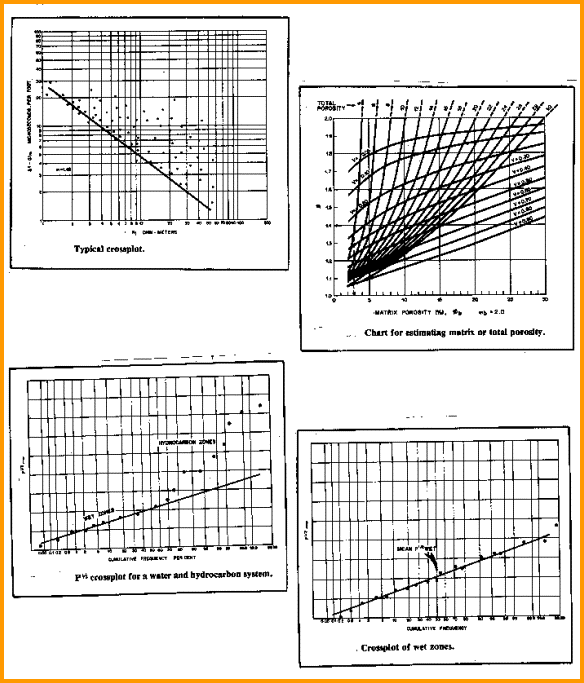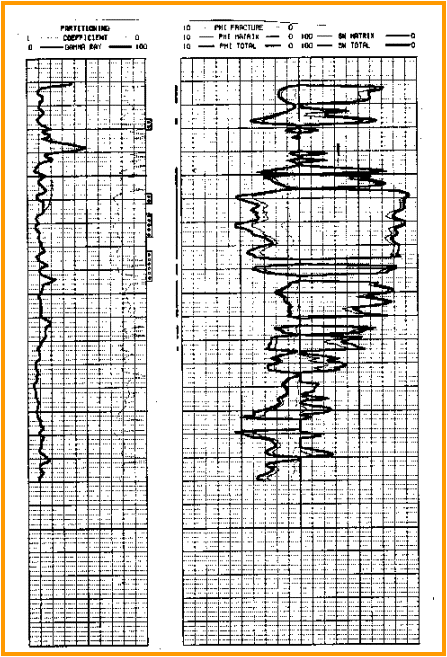Water Saturation in the Dual Porosity Model
The average water saturation of the composite system is calculated using a statistical parameter, P, originally introduced to the oil industry by Porter, Pickett, and Whitman. Empirically, it has been found that P has a normal distribution for intervals which are 100 per cent saturated with water. Intervals with some hydrocarbon saturation deviate from the normal distribution.

 Calculating P For sonic logs:     15a: P = (RESD * (DELT - DELTMA) ^ Md) ^ (1/2) For density logs:     15b: P = (RESD * (DENS - DENSMA) ^ Md) ^ (1/2) If effective porosity has been computed from density neutron crossplot:     15c: P = (RESD * (PHIe ^ Md) ^ (1/2)

WHERE:
DELT = sonic log reading (usec/ft or usec/m)
DELTMA = sonic matrix transit time (usec/ft or usec/m)
DENS = density log reading (g/cc or kg/m3)
DENSMA = matrix density (g/cc or kg/m3)
Md = cementation exponent for double porosity model, found from a Pickett plot
P = statistical parameter (unitless)
PHIe = effective porosity of double porosity system (fractional)
RESD = deep resistivity log reading (ohm-m)Pickett plot, partition factor, and probability graphs for Aguilera’s dual porosity model

This illustration, bottom left, shows a schematic of P vs cumulative frequency on probability paper for a water and hydrocarbon system. The 100 per cent water saturated zones form a straight line, and the hydrocarbon intervals deviate from this line and are thus easily recognized.

Bottom right shows the same type of plot for only the water bearing intervals. The mean value of P for water zone, Pmean, is determined from this graph at a cumulative frequency of 50 per cent. An arithmetic average of the P values from the water leg is usually satisfactory, so the probability plot is not necessary.

Having the mean value of P from the water zone allows us to calculate the water saturation of the dual porosity system from the following.

 Partitioning water saturation     16: Swd = (Pwtr / Phyd) ^ (1/N)     17: Swf = (VISW * WOR) / (Bo * VISO + VISW * WOR)     18: Swe = (Swd - V * Swf) / (1 - V)

Where:
Bo = oil formation volume factor (vol/vol)
N = water saturation exponent (unitless)
Phyd = parameter P for each hydrocarbon zone (unitless)
Pwtr = mean value of P for water bearing intervals (unitless)
Swd = water saturation for the double porosity system (fractional)
Swe = water saturation for the matrix rock (fractional)
Swf = water saturation for the fracture (fractional)
VISW = water viscosity (cp)
VISO = oil viscosity (cp)
WOR = water/oil ratio, (vol/vol)

This long evaluation process requires the reading, plotting, and crossplotting of large volumes of data, and requires a large number of calculations. This makes it an ideal computer application and hand calculation is not recommended. A solution for a gas reservoir was never published. In many cases Swf is assumed to be 0.0 (because WOR = 0) so this assumption can be used for gas wells also.

NOTE: This is essentially an "Rwa type" saturation method and relies on the presence of a water zone. In the absence of a water zone, an rchie water saturation solution (Swa) will have to suffice, giving the equivalent of Swe. Swf is not derived from log data. If parameters are unknown, start with VISW =1.0, VISO = 2.0, WOR = 0.0, and Bo = 0.8. This makes Swf = 0.0 during production, which is close to the truth.

 Standard Archie solution     19: Swa = (A * RW@FT / (PHIe ^ Md) / RESD) ^ (1/N)

Where:
A = tortuosity exponent (unitless)
Md = cementation exponent for matrix rock with fractures (unitless)
N = saturation exponent (unitless)
PHIe = effective porosity (fractional)
RESD = deep resistivity log reading (ohm-m)
RW@FT = water resistivity at formation temperature (ohm-m)
Swa = effective water saturation (fractional)

RECOMMENDED PARAMETERS:
If zone is not heavily fractured:
For sandstones:
A = 0.62
Mb = 2.15
N = 2.00

For carbonates:
A = 1.00
Mb = 2.00
N = 2.00

If zone is fractured:
Mb = 1.4 to 2.0
A = 1.00
N = 2.00

NOTE: The symbol M is used elsewhere in this book as the cementation exponent for the Archie equation. Mb is used here to indicate the use of Archie for the fractured reservoir case.Dual porosity model results in a Williston Basin well

The log  shows the computed log results for a Williston Basin Mississippian fractured zone. The contribution of fracture related porosity is about 1% porosity, but water saturation is 5 to 10% lower than the analysis without the fractures. The partitioning coefficient varies and was solved by iteration.

Page Views ---- Since 01 Jan 2015
Copyright 2023 by Accessible Petrophysics Ltd.
CPH Logo, "CPH", "CPH Gold Member", "CPH Platinum Member", "Crain's Rules", "Meta/Log", "Computer-Ready-Math", "Petro/Fusion Scripts" are Trademarks of the Author